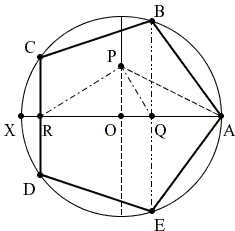# Construction of Regular Pentagon by H. W. Richmond

As Ptolemy's construction described by S. Brodie, the one below seeks to construct a regular pentagon inscribed in a given circle. The latter is dated 1893 and attributed to H. W. Richmond. The approach has been expanded by [Conway and Guy] to the construction of other regular polygons.Let XA be a diameter of the circle with center O.

1. Choose P midway on the radius perpendicular to XA.

2. Draw a bisector of ∠APO to the intersection Q with XA,

3. and an external bisector to the intersection with XA at R.

4. If A is taken to be one of the vertices of the regular pentagon, Q and R are the projections of the other four onto XA. These can be obtained by erecting perpendiculars to XA at Q and R.

### Proof

Assume the radius of the circle is 1. Then OP = 1/2. From the Pythagorean theorem, AP = 5/2. By a property of angle bisectors,

OQ / AQ = OP / AP = 1/2 : 5/2.

Since OQ + AQ = 1, we find OQ = (5 - 1) / 4. But the latter is the value of cos(72°), which allows us to conclude (from ΔOBQ) that ∠BOQ = 72°, such that A and B are indeed successive vertices of a regular pentagon.

Similarly, but using a property of external angle bisectors, OR = (5 + 1) / 4, which is cos(36°). So that ∠COR = 36° and C is the next (after B) vertex of the regular pentagon.

(This construction is easily Regular Pentagon Inscribed in Circle by Paper Foldingimplementable by paper folding.)

### References

1. J. H. Conway, R. Guy, The Book of Numbers, Springer, 1996